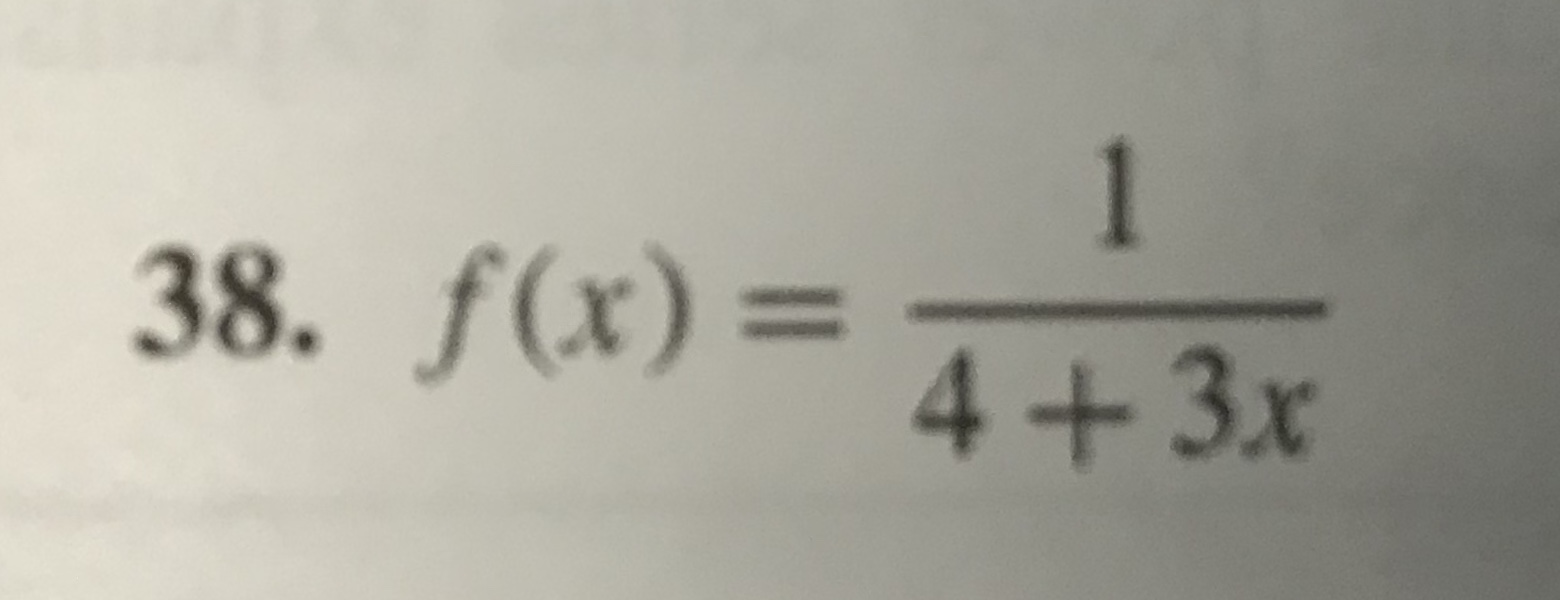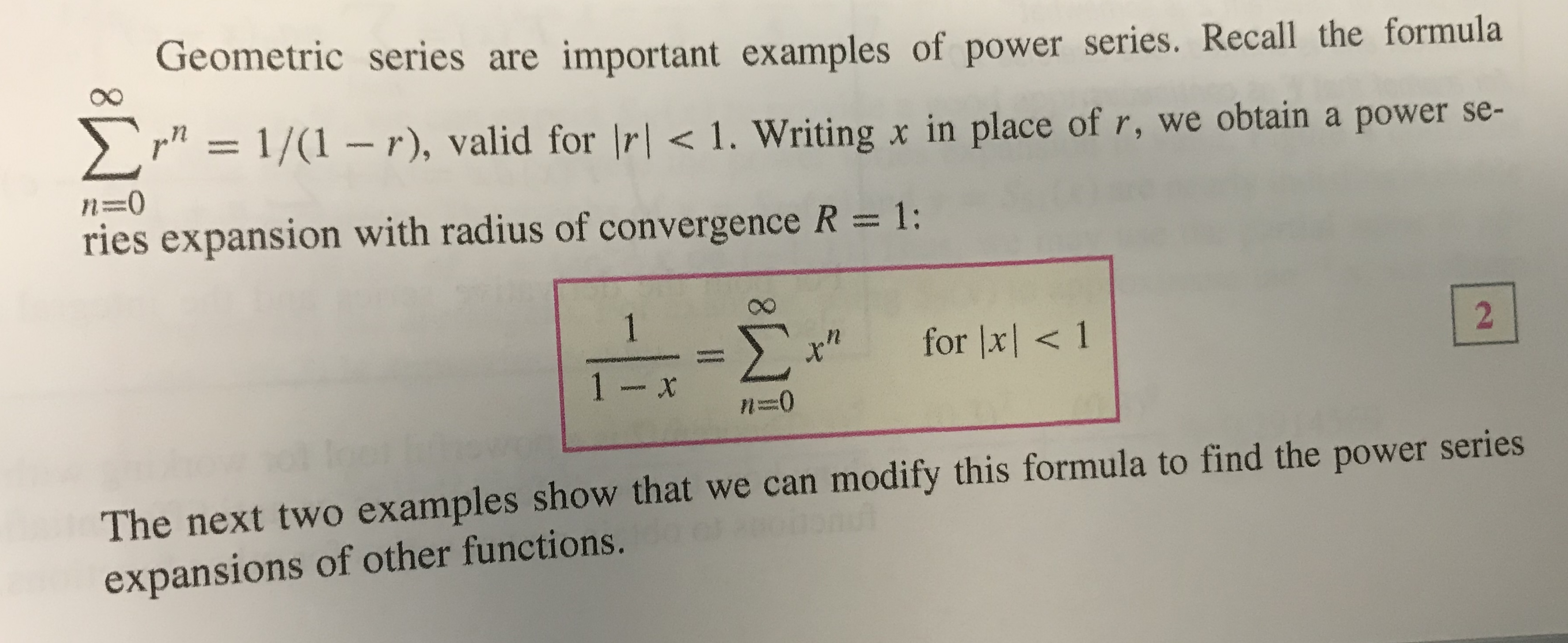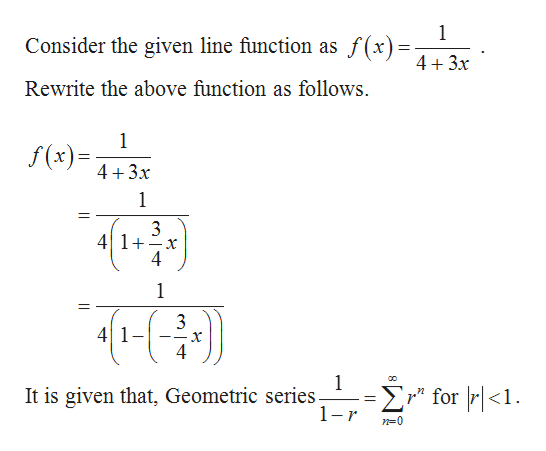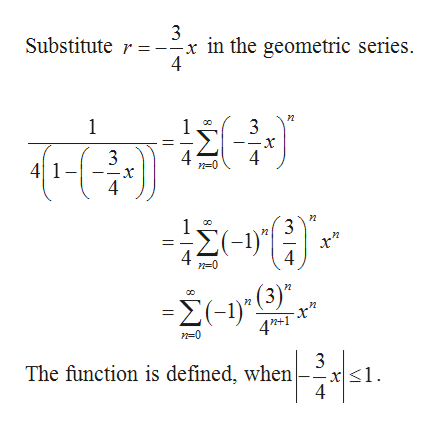# 138. f(x)=4+3x Geometric series are important examples of power series. Recall the formulaΣ= 1/(1- r), valid for Ir

Question
10 views

For #38, use Equation 2 to expand the function in a power series with center c=0 and determine the interval of convergence.help_outlineImage Transcriptionclose1 38. f(x)= 4+3x fullscreenhelp_outlineImage TranscriptioncloseGeometric series are important examples of power series. Recall the formula Σ = 1/(1- r), valid for Ir<1. Writing x in place of r, we obtain a power se- n-0 ries expansion with radius of convergence R = 1: 1 Σ for x<1 2 1 - x n=0 venetas The next two examples show that we can modify this formula to find the power series expansions of other functions. fullscreen
check_circle

Step 1help_outlineImage Transcriptionclose1 Consider the given line function as f(x) = 4+3.x Rewrite the above function as follows 1 f(x) 4 3x 1 41 x 1 3 4 1 1 -Σ' for r | <1. 1 It is given that, Geometric series. r n-0 fullscreen
Step 2help_outlineImage TranscriptioncloseSubstitute r in the geometric series 4 1 3 41- 4 4 4 - 3 Σ1)ν (3)" -Σ). 4*+I 3 The function is defined, when fullscreen

### Want to see the full answer?

See Solution

#### Want to see this answer and more?

Solutions are written by subject experts who are available 24/7. Questions are typically answered within 1 hour.*

See Solution
*Response times may vary by subject and question.
Tagged in

### Other